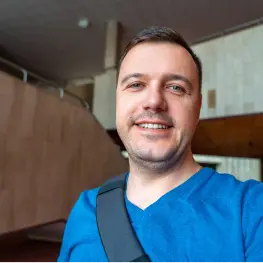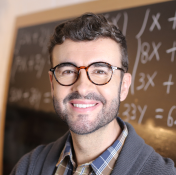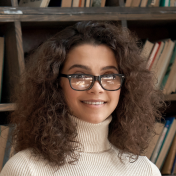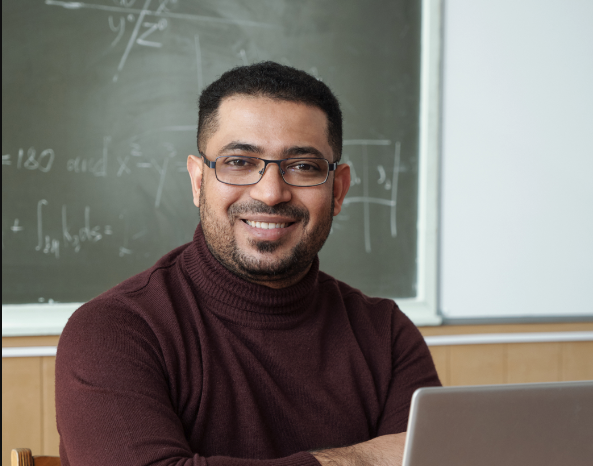# Trust the nation's largest network for Probability tutors

## Find a great match with ourGood Fit Guarantee

#### Featured by the nation’s most respected news sources#### Tutors from top universitiesGet 1:1 Help Fast

# The Best Probability Tutors in Wilmington, NC

## Find the best local Probability tutor in WilmingtonRyan N.
80/hour

### Math/Stat tutoring from algebra to abstract topics and proof-writing.

Probability theory is the mathematical field that I have probably spent the most time studying and tutoring, and I very much enjoy helping people reorient their intuition to overcome the difficulties that nearly everyone experiences... See Ryan's full profile

5.0 (1337)
80/hour
4,684 hours tutoring

Probability theory is the mathematical field that I have probably spent the most time studying and tutoring, and I very much enjoy helping people reorient their intuition to overcome the difficulties that nearly everyone experiences...See Ryan's full profileEric T.
66/hour

### Mathematics is the language of the universe

I have tutored students in Probability and Statistics 1 and Probability and Statistics 2 at Eastern Michigan University in the Math Lab as an employee.I have a M.A. in Mathematics and Statistics from Eastern Michigan University with... See Eric's full profile

4.9 (1072)
66/hour
3,815 hours tutoring

I have tutored students in Probability and Statistics 1 and Probability and Statistics 2 at Eastern Michigan University in the Math Lab as an employee.I have a M.A. in Mathematics and Statistics from Eastern Michigan University with...See Eric's full profileKiran P.
75/hour

### Math Ph.D. With Expertise in Finite Math

., I am extremely comfortable with the topics in a finite math course - linear equations, matrices, linear programming, and elementary probability and counting problems. In my many years of tutoring and classroom teaching, I’ve taught... See Kiran's full profile

5.0 (110)
75/hour
198 hours tutoring

., I am extremely comfortable with the topics in a finite math course - linear equations, matrices, linear programming, and elementary probability and counting problems. In my many years of tutoring and classroom teaching, I’ve taught...See Kiran's full profileTessa W.
75/hour

### Math PhD with Over 20 Years Experience, Patient and Kind

...Precalculus, Business Calculus, Calculus (all flavors, including AP AB/BC, and Calculus I, II & III), Intro to Statistics, Probability and Statistics, AP Statistics, Differential Equations, Linear Algebra, and Numerical Analysis. I also tutor ACT and SAT... See Tessa's full profile

5.0 (356)
75/hour
1,242 hours tutoring

...Precalculus, Business Calculus, Calculus (all flavors, including AP AB/BC, and Calculus I, II & III), Intro to Statistics, Probability and Statistics, AP Statistics, Differential Equations, Linear Algebra, and Numerical Analysis. I also tutor ACT and SAT...See Tessa's full profileWei C.
200/hour

### Experienced Math/Physics PhD with Patience and Humor

I have a BS and PhD in Physics. I have high scores in many discrete math courses, like probability, combinatorics, graph theory. Discrete math is used in many physics applications, especially statistical physics. See Wei's full profile

5.0 (206)
200/hour
724 hours tutoring

I have a BS and PhD in Physics. I have high scores in many discrete math courses, like probability, combinatorics, graph theory. Discrete math is used in many physics applications, especially statistical physics. See Wei's full profileSuzan O.
75/hour

### Probability Teacher Specializing in Statistics

I have tutored Statistics and Probability for about two years. I can help with probability concepts, math, and combinations/permutations. I can also teach probability in the context of statistical analyses. See Suzan's full profile

5.0 (289)
75/hour
990 hours tutoring

I have tutored Statistics and Probability for about two years. I can help with probability concepts, math, and combinations/permutations. I can also teach probability in the context of statistical analyses.See Suzan's full profileAJ N.
50/hour

### Calculus, Linear Algebra, Probability, Real Analysis, P, FM, IFM, SRM

Hi! I have taken many graduate level courses in mathematics including real and complex analysis, topology, differential geometry, differentiable manifolds, commutative algebra, algebraic geometry, and topology. I hold a Bachelors of Science in... See AJ's full profile

5.0 (254)
50/hour
1,004 hours tutoring

Hi! I have taken many graduate level courses in mathematics including real and complex analysis, topology, differential geometry, differentiable manifolds, commutative algebra, algebraic geometry, and topology. I hold a Bachelors of Science in...See AJ's full profile100/hour

### 15 year teacher of AP Stats, probability is my jam!

Probability as a subset of AP Statistics and standard algebra curriculum is essentially understanding basic counting principles and identifying a few archetype problems. I've taught AP Stats for 15 years and was invited to develop the... See Adam's full profile

5.0 (197)
100/hour
721 hours tutoring
Response Time: 17 minutes
Note that average response times may vary depending on a variety of factors, including time of day.

Probability as a subset of AP Statistics and standard algebra curriculum is essentially understanding basic counting principles and identifying a few archetype problems. I've taught AP Stats for 15 years and was invited to develop the...See Adam's full profileYvan M.
80/hour

### Five-star Math Tutor, 10+ years experience.

I've been helping students succeed in math since 2010. I started tutoring in high school and very quickly fell in love with teaching. I love seeing the excitement build in my students as they not only begin to understand, but also enjoy a subject... See Yvan's full profile

5.0 (275)
80/hour
757 hours tutoring
Response Time: 8 minutes
Note that average response times may vary depending on a variety of factors, including time of day.

I've been helping students succeed in math since 2010. I started tutoring in high school and very quickly fell in love with teaching. I love seeing the excitement build in my students as they not only begin to understand, but also enjoy a subject...See Yvan's full profileRyan O.
70/hour

### PhD in Mathematics with nearly 20 years Teaching Experience

Probability is one of my favorite branches of mathematics and science. I have taught and tutored different levels of probability and statistics for nearly 20 years. These courses range from elementary courses (no calculus required)... See Ryan's full profile

4.9 (62)
70/hour
255 hours tutoring

Probability is one of my favorite branches of mathematics and science. I have taught and tutored different levels of probability and statistics for nearly 20 years. These courses range from elementary courses (no calculus required)...See Ryan's full profileEthan G.
125/hour

### Experienced Math and Physics Tutor

I have a master's degree in mathematics and love to teach. I have been tutoring math and physics students since 2012. Both of these subjects can be pretty tough, and I am passionate about helping students to really understand and succeed at them.... See Ethan's full profile

5.0 (1110)
125/hour
4,556 hours tutoring

I have a master's degree in mathematics and love to teach. I have been tutoring math and physics students since 2012. Both of these subjects can be pretty tough, and I am passionate about helping students to really understand and succeed at them....See Ethan's full profileLisa O.
140/hour

### Caltech Graduate, Experienced Probability Tutor

...calculating the probability of A and B occurring, or A or B occurring; conditional probability; definition of factorial, combination, permutation; expected value, variance, standard deviation; probability density functions, probability mass functions... See Lisa's full profile

5.0 (1556)
140/hour
4,362 hours tutoring

...calculating the probability of A and B occurring, or A or B occurring; conditional probability; definition of factorial, combination, permutation; expected value, variance, standard deviation; probability density functions, probability mass functions...See Lisa's full profileTaylor K.
96/hour

### Experienced, Certified H.S. Math Teacher

I have four years experience teaching high school mathematics full-time, and I have taught Algebra 1, Algebra 2, Geometry, and AP Statistics during this time. In my Geometry and AP Stats courses, I give a thorough treatment of probability... See Taylor's full profile

5.0 (369)
96/hour
966 hours tutoring

I have four years experience teaching high school mathematics full-time, and I have taught Algebra 1, Algebra 2, Geometry, and AP Statistics during this time. In my Geometry and AP Stats courses, I give a thorough treatment of probability...See Taylor's full profileK.J. P.
80/hour

### Math tutor in Hudson,Ohio. You won't find better.

I love math. I love teaching it. I find it intrinsically satisfying to see the light come on in a student's eyes. My first math lesson that I taught was when I was 5. My neighbor, still in diapers, kept skipping 4 when counting from 1 to 10.... See K.J.'s full profile

4.9 (1428)
80/hour
4,206 hours tutoring
Response Time: 14 minutes
Note that average response times may vary depending on a variety of factors, including time of day.

I love math. I love teaching it. I find it intrinsically satisfying to see the light come on in a student's eyes. My first math lesson that I taught was when I was 5. My neighbor, still in diapers, kept skipping 4 when counting from 1 to 10....See K.J.'s full profileAlex S.
120/hour

### Ph.D., years of teaching and research experience

Ph.D. in Physics; years of teaching and research experience in areas such as quantum physics, statistical mechanics, quantum information theory. Statistics and probability theory are in the core of these and many other areas of physics. See Alex's full profile

4.9 (442)
120/hour
1,691 hours tutoring
Response Time: 16 minutes
Note that average response times may vary depending on a variety of factors, including time of day.

Ph.D. in Physics; years of teaching and research experience in areas such as quantum physics, statistical mechanics, quantum information theory. Statistics and probability theory are in the core of these and many other areas of physics.See Alex's full profileRebecca V.
110/hour

### Extensive experience in Probability

From teaching classes such as geometry and AP Statistics, I have 8+ years of teaching probability concepts. A difficult subject for a lot of students, but a very important one to understand. I really enjoy teaching the "why"... See Rebecca's full profile

5.0 (7)
110/hour
25 hours tutoring

From teaching classes such as geometry and AP Statistics, I have 8+ years of teaching probability concepts. A difficult subject for a lot of students, but a very important one to understand. I really enjoy teaching the "why"...See Rebecca's full profileLauri L.
175/hour

### 5 Star Math Tutor - Experienced, Knowledgeable and Patient

I’m an experienced classroom teacher and tutor and I can help you be more successful in AP Statistics and Algebra and improve your score on the ACT or SAT. I’ve taught AP Stat for 15 years and I’ve graded the AP exam for the College Board... See Lauri's full profile

5.0 (559)
175/hour
1,700 hours tutoring

I’m an experienced classroom teacher and tutor and I can help you be more successful in AP Statistics and Algebra and improve your score on the ACT or SAT. I’ve taught AP Stat for 15 years and I’ve graded the AP exam for the College Board...See Lauri's full profileKubrat D.
175/hour

### Ivy League expert in Calculus, Probability and Linear Algebra

I am a Berkeley/Princeton educated tutor with 10 years of experience teaching all college math subjects. Unlike a lot of other tutors, I have the educator mindset rather than the student mindset, and I can break down complicated math concepts down... See Kubrat's full profile

5.0 (456)
175/hour
1,659 hours tutoring

I am a Berkeley/Princeton educated tutor with 10 years of experience teaching all college math subjects. Unlike a lot of other tutors, I have the educator mindset rather than the student mindset, and I can break down complicated math concepts down...See Kubrat's full profileRoger R.
88/hour

### Expert Calculus and Linear Algebra Tutorials

Subjects: Calculus (I-IV, AP), Real and Complex Analysis, Linear Algebra, Ordinary Differential Equations, Probability, Statistics, Discrete/Finite Mathematics, IB Mathematics, Algebra I/II, Trigonometry, Precalculus, Geometry... See Roger's full profile

5.0 (18)
88/hour
67 hours tutoring

Subjects: Calculus (I-IV, AP), Real and Complex Analysis, Linear Algebra, Ordinary Differential Equations, Probability, Statistics, Discrete/Finite Mathematics, IB Mathematics, Algebra I/II, Trigonometry, Precalculus, Geometry...See Roger's full profileJoshua L.
120/hour

### Ph.D. Student in Physics with a Focus on Probability

Even though I argue linear algebra is the most important field of mathematics right now, I also argue probability theory will be the most important field of mathematics in our future! I am a Ph.D. student in theoretical physics, I have seen... See Joshua's full profile

4.9 (92)
120/hour
317 hours tutoring

Even though I argue linear algebra is the most important field of mathematics right now, I also argue probability theory will be the most important field of mathematics in our future! I am a Ph.D. student in theoretical physics, I have seen...See Joshua's full profileSeh-kai L.
115/hour

### Your personal Sehkaiatrist (but for math)!

I am able to tutor all math subjects including, but not limited to: algebra 1/2, geometry, pre-calculus, trigonometry, calculus 1/2/3, differential equations, linear algebra, real analysis, abstract algebra, and probability.... See Seh-kai's full profile

5.0 (120)
115/hour
349 hours tutoring
Response Time: 9 minutes
Note that average response times may vary depending on a variety of factors, including time of day.

I am able to tutor all math subjects including, but not limited to: algebra 1/2, geometry, pre-calculus, trigonometry, calculus 1/2/3, differential equations, linear algebra, real analysis, abstract algebra, and probability....See Seh-kai's full profileRaphael L.
139/hour

### Harvard PhD in statistical physics, great at explaining statistics!

I'm excellent at probability and statistics; I was top 50 in the US Math Olympiad and am good with tricky probability questions. I tutor from elementary statistics and AP statistics to selected graduate level classes in the... See Raphael's full profile

5.0 (1725)
139/hour
5,767 hours tutoring

I'm excellent at probability and statistics; I was top 50 in the US Math Olympiad and am good with tricky probability questions. I tutor from elementary statistics and AP statistics to selected graduate level classes in the...See Raphael's full profileNguyen N.
75/hour

### Experienced teacher, here to help!

I have a PhD in Mathematics, and have taught college math courses for more than 10 years ranging from computational courses to more theory-based courses. I can help you with topics in intro courses like calculus (single and multivariable), linear... See Nguyen's full profile

5.0 (210)
75/hour
520 hours tutoring

I have a PhD in Mathematics, and have taught college math courses for more than 10 years ranging from computational courses to more theory-based courses. I can help you with topics in intro courses like calculus (single and multivariable), linear...See Nguyen's full profileCasey F.
97/hour

### Passionate High School and College Math Tutor

I graduated from MIT with degrees in Math and Physics. I also hold a PhD degree in Pure Math at UCLA. The most rewarding part of teaching is seeing students' improvement. I TA'ed for undergraduate math classes at UCLA for four years. SAT/ACT... See Casey's full profile

5.0 (217)
97/hour
571 hours tutoring

I graduated from MIT with degrees in Math and Physics. I also hold a PhD degree in Pure Math at UCLA. The most rewarding part of teaching is seeing students' improvement. I TA'ed for undergraduate math classes at UCLA for four years. SAT/ACT...See Casey's full profileJodi M.
120/hour

### I can make statistics understandable.

I specialize in undergraduate probability and statistics classes as well as MBA statistics courses. With a masters degree in a statistics related field (econometrics) and hundreds of hours of experience helping college and grad school... See Jodi's full profile

5.0 (1485)
120/hour
3,630 hours tutoring

I specialize in undergraduate probability and statistics classes as well as MBA statistics courses. With a masters degree in a statistics related field (econometrics) and hundreds of hours of experience helping college and grad school...See Jodi's full profile

### Trusted with over6 million hoursof lessons since 2005Success stories

#### Real stories from real people

Since 2005, Wyzant has provided a way for people to learn any subject in a way that works for them.

##### Grace M.

18 Probability lessons

We covered topics such as conditional probability, counting rules, events, discrete random variable, binomial probability, hypergeometric probability, normal distribution/standard normal distribution, calculating probability using normal table, how to use a T table, normal approximation to the binomial probability, sampling theory, estimation/ statistical test of hypothesis, tests about a population mean, tests for difference between two populations, and test for correlation.
##### Darin B.

1 Probability lesson

Jason, helped me with college level Probability. The level of Probabilities included but not limited to: Distinguish simple and theoretical probability from classical probability theory, joint and conditional probabilities, probability mass function, measure a probability distribution, describe a binomial distribution, calculate Z scores for a normal distribution and calculate the standard error of a sample.
##### Amanda K.

2 lessons

Statistics • Review of computations for arithmetic mean, standard deviation, and variance of a sample • Computation of quadratic mean and harmonic mean of a data set • Computation of the sample proportion • Use of the normal distribution to compute a confidence interval for a sample mean • Compuation of the margin of error for a sample mean • Use of the Student's t-distribution to compute a confidence interval for a sample mean • Computation of the number of degrees of freedom when using the t-statistic or the Chi-squared distribution • Determination of critical values when using the normal distribution, t-distribution, or Chi-squared distribution to compute confidence intervals • Use of tables for the normal, Student t, and Chi-square distributions • Probability computations using classical probability theory, i.e. number of successful outcomes divided by the number of possible outcomes • Probability of consecutive independent events (product of individual probabilities) • Binomial experiment criteria, and computation of binomial probability • Approximating the binomial distribution using the normal distribution
How much for private Probability tutoring lessons

## Wilmington Probability Tutors Cost \$35 - 65 per hour on average

What’s a fair price for a private Wilmington Probability tutor? Wilmington Probability tutors using Wyzant are professional subject experts who set their own price based on their demand and skill.

\$25\$100
\$35
\$65

Compare tutor costs. With a range of price options, there’s a tutor for every budget.

No Upfront Fees

No Costly Packages

Only pay for the time you need. Whether it’s one lesson or seven, you decide what to spend.

### Love Your Lesson Or It’s Free

Your first hour with a new tutor is protected by Wyzant’s Good Fit Guarantee. If you’re not satisfied with your lesson, you don’t pay. No questions asked.

### Reported on by leading news outlets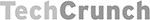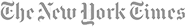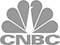### Find Wilmington Private Tutors in Subjects related to Probability

Wyzant makes it easy to get lessons from private tutors in any subject related to Probability. Choose your Wilmington tutor by reading ratings and reviews as well as comparing the rates of tutors on Wyzant who are subject-matter experts prepared to teach you in a wide variety of topics:

### Probability Tutoring is just the beginning...

Tap into Wyzant’s digital nationwide network to find either a local or online tutor for subjects related to Probability. Find the perfect tutor for you in over 250 subjects and test prep areas including:

### Tutoring Near Wilmington

Find a local tutor in areas near Wilmington. Our nationwide network of private tutors makes it easy to find an instructor nearby. Learn a new job skill or ace the test. Compare tutor costs and qualifications and find the ideal tutor for you today.

### Find Wilmington tutors

Want to browse local tutors near you in more subjects? See all tutors in Wilmington

### Find online Probability tutors

Get Probability help anytime! We only accept the best online Probability tutors into our community. Get the help you need, right when you need it with the convenience of online lessons.